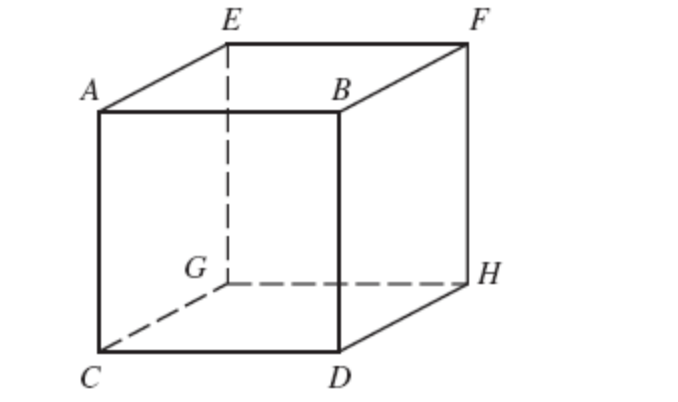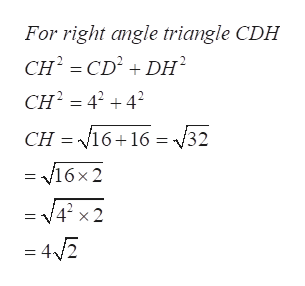EВCHI

Question

If the length of each edge of the cube shown in the figure is 4 inches, find the following.

(a) the length of diagonal CH.
CH =

in

(b) the length of diagonal CF.
CF =

inhelp_outlineImage TranscriptioncloseE В C HI fullscreen
Step 1

Refer to the diagram in the question,

As mentioned the edge of the cube is 4 inches and we know that all the sides of the cube are equal. ...help_outlineImage TranscriptioncloseFor right angle triangle CDH CH CD2DH CH44 CH 16 16 32 = v16x2 = V42 x 2 =4Z fullscreen

Want to see the full answer?

See Solution

Want to see this answer and more?

Our solutions are written by experts, many with advanced degrees, and available 24/7

See Solution
Tagged in

Other